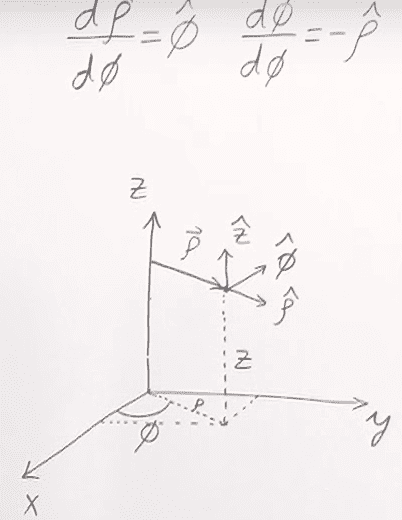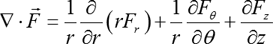# Del operator in a Cylindrical vector fucntion

• I
Hi there
1. I'm having a hard time trying to understand how come ∂r^/∂Φ = Φ^ ,∂Φ/∂Φ = -r^ -> these 2 are properties that lead to general formula.
2. I've been thinking about it and I couldn't explain it. I understand every step of "how to get Divergence of a vector function in Cylindrical Coordinates" except for these formulas below.A picture attached for visualization.
3.General formula
Thanks for any help.

#### Attachments

tnich
Homework Helper
Hi there
1. I'm having a hard time trying to understand how come ∂r^/∂Φ = Φ^ ,∂Φ/∂Φ = -r^ -> these 2 are properties that lead to general formula.
2. I've been thinking about it and I couldn't explain it. I understand every step of "how to get Divergence of a vector function in Cylindrical Coordinates" except for these formulas below.
View attachment 226837 A picture attached for visualization.
3.
View attachment 226838 General formula
Thanks for any help.
If you apply the definition of derivative, these formulas will fall right out. For ##\frac {\partial \hat r} {\partial \theta}##, start with ##\hat r = (cos \theta, sin \theta)##.
By the way, you can easily write ##\hat r## as ##\#\#\backslash hat~r\#\###. For more cool formatting tricks, see the LaTex tutorial.